Make Faber Castell, Germany Model 67/38 Tachymeter 400g Notes1. This rule is obviously aimed at a specialist surveying market. 2. The rule is a simplex one with reversible slide. With the slide in its "front" position the arrangement of scales is fairly normal except for the position of the trig scales on the lower part of stock normally reserved for inch or centimetre scales and the fact that they use "grads" (where the circle is divided into 400 grads rather than 360 degrees). 3. The cursor has two uncommon features. (i)  The first is a line at 683 on the A scale. This is used for "distance at sea" calculations in the formula:      h = 0.0683 d2      where:      h = is height above sea level in metres      d = is distance to the horizon in kilometres. It takes account of both refraction and the curvature of the earth.     (ii) The second is a vernier scale on the centimetre rule part of the slide rules. This is shown in detail below. The long red line to the left is almost half way between 6.2 and 6.3 cm. The short red line which most closely corresponds to one of the black markings is the fourth from the left. The value is therefore 6.24. (Thanks to members of the slide rule group for the above information) 4. The reverse of the slide most unusual. It has the following features: Vertical lines at each end to align the slide with the stock (The slide has to be turned - there are no alignment marks on the back of the stock.) A scale marked 1 - cos2 . This can be seen most clearly at the detail of the right hand end of the slide. The values of 20.5g aligns with .10 on the A scale. Cos of 20.5 g is 0.9486 and 1 - 0.94862 is equal to 0.1. Underneath it is an unmarked scale which runs from 2 to 7. This a scale of sine measured in grads. A scale marked sin . cos. This runs from 6 to 22 grad in the left centre of the slide and 0.6 to 7 grads on the bottom edge of the slide. The image of the centre of the slide shows the value of 22 grad. Sin 22 g is 0.339 and cos 22 g is 0.941, the product of these two is .319, which corresponds to the value on the D scale. A scale marked cos2 . This can be seen as a short scale on the right of slide. It runs from 0 to 25 g. The value of cos 25 g is .924 and .924 squared is 0.854, which is the corresponding value on the A scale. 5. I bought this rule at the same time as a Unique Navigational rule so they were probably both owned the same person. Front view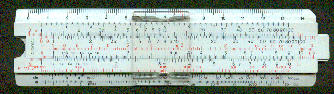Detail - front left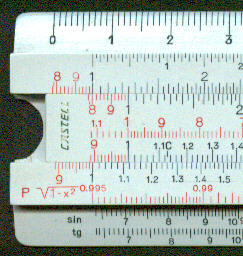Detail - cursor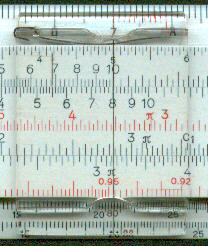Detail -front right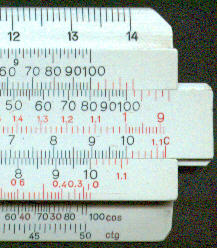Detail - left endDetail - centre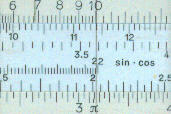Detail - right end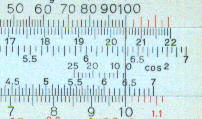Detail - vernier scale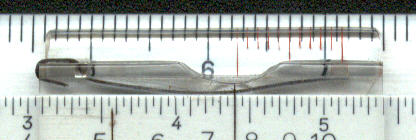Manufacturing date 1953 (marked on rule) Length 5" Material Plastic Scales A, [B, CI, C/ 1 - cos2, sin.cos, cos2] D, P, S, T Cursor Plastic Hair lines Two. Documentation None Condition Good.